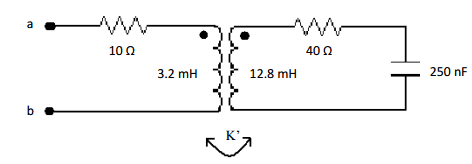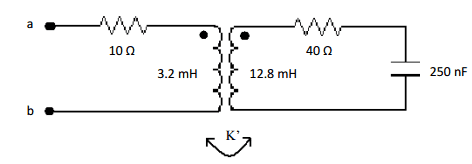# Linear transformer and coupling coefficient

Rombus

## Homework Statement

The coupoing coefficient of K' of a linear transformer is adjusted so that Zab is purely reissitive when ω= 25k rad/s. What is Zab?Not sure

## The Attempt at a Solution

I'm not sure how to being this problem. For one, from what I've been reading I'm not sure how the connection between frequency and Zab is made. Also, I'm not sure what the question is asking for by 'purely resistive'. Does this mean there is no comblex part?

I'm pretty sure I understand the coupling coefficient. I'm not sure how to apply it to this problem.

Mentor

## Homework Statement

The coupoing coefficient of K' of a linear transformer is adjusted so that Zab is purely reissitive when ω= 25k rad/s. What is Zab?Not sure

## The Attempt at a Solution

I'm not sure how to being this problem. For one, from what I've been reading I'm not sure how the connection between frequency and Zab is made. Also, I'm not sure what the question is asking for by 'purely resistive'. Does this mean there is no comblex part?

I'm pretty sure I understand the coupling coefficient. I'm not sure how to apply it to this problem.

Yes, the "purely resistive" part means that the imaginary part of the complex impedance is zero at that frequency.

Write the equation for the complex input impedance as a function of ω, including the turns ratio and the coupling coefficient. Then set the frequency as instructed, and solve for the value of K' that will cause the imaginary part of the impedance to go to zero...

Rombus

So if my equation is:

$$\bf{Z}ab R_1 + j \omega L_1 + \frac { \omega^2 (k ( \sqrt{L_1 L_2)^2} {R_2 + \frac{1} {j \omega C}} + j \omega L_2)}$$

I solve for k, the coupling coefficent so that the imaginary part of the impedence is zero?

Is K' the same as k? Or is K' my mutual inductance that I find?

Edit: Not sure why my code isn't displaying correctly.

Last edited: